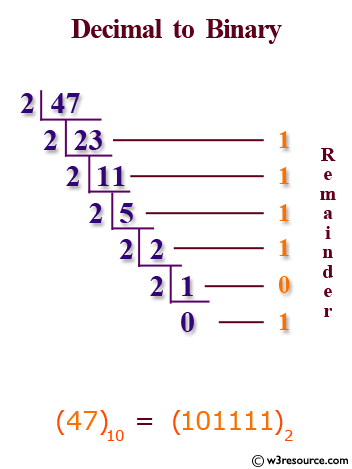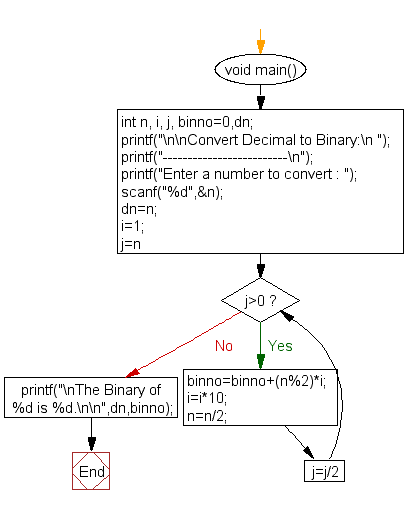﻿ C : Convert decimal number to binary without using an array# C Exercises: Convert a decimal number to binary without using an array

## C For Loop: Exercise-41 with Solution

Write a program in C to convert a decimal number into binary without using an array.

Pictorial Presentation:Sample Solution:

C Code:

``````#include <stdio.h>
#include <stdlib.h>
char *decimal_to_binary(int);
char *decimal_to_binary(int dn)
{
int i, j, temp;
char *ptr;
temp = 0;
ptr = (char*)malloc(32+1);
for (i = 31 ; i >= 0 ; i--)
{
j = dn >> i;
if (j & 1)
*(ptr+temp) = 1 + '0';
else
*(ptr+temp) = 0 + '0';
temp++;
}
*(ptr+temp) = '\0';
return  ptr;
}
int main()
{
int dn;
char *ptr;
printf("Input a decimal number: ");
scanf("%d", &dn);
ptr = decimal_to_binary(dn);
printf("Binary number equivalent to said decimal number is: %s", ptr);
free(ptr);
return 0;
}
```
```

Sample Output:

```Input a decimal number: 25
Binary number equivalent to said decimal number is: 0000000000000000000000000001
1001
--------------------------------
Process exited after 3.388 seconds with return value 0
Press any key to continue . . .
```
```Input a decimal number: 105
Binary number equivalent to said decimal number is: 0000000000000000000000000110
1001
--------------------------------
Process exited after 5.013 seconds with return value 0
Press any key to continue . . .
```
```Input a decimal number: 1105
Binary number equivalent to said decimal number is: 0000000000000000000001000101
0001
--------------------------------
Process exited after 17.33 seconds with return value 0
Press any key to continue . . .
```

Flowchart:C Programming Code Editor:

Improve this sample solution and post your code through Disqus.

What is the difficulty level of this exercise?

Test your Programming skills with w3resource's quiz.

﻿